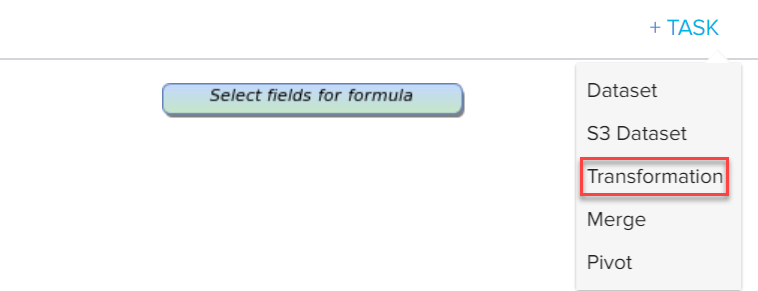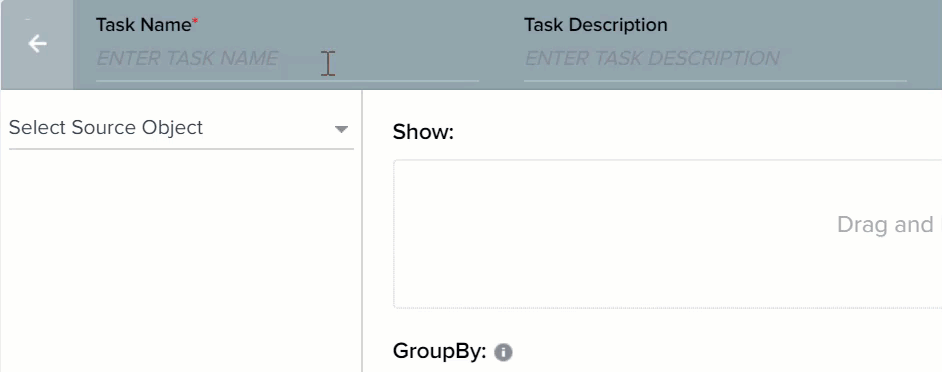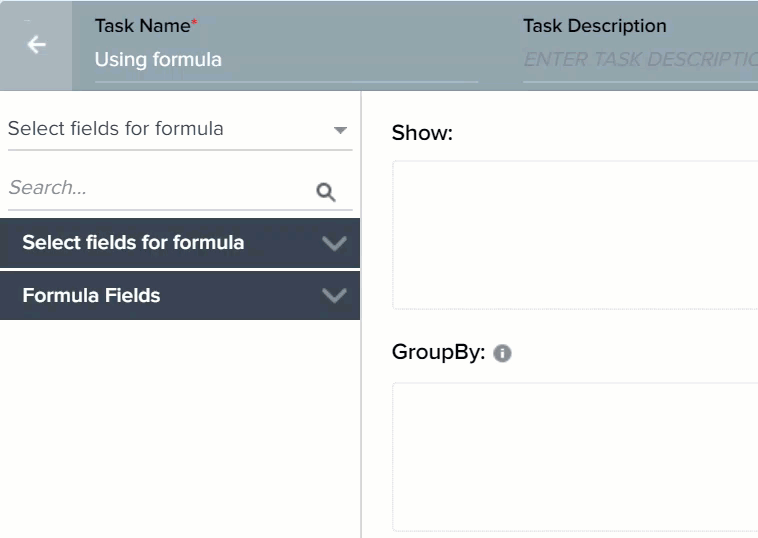Skip to main content

# Formula Fields in Rules Engine

`Gainsight NXT`
Gainsight helps innovative companies protect and nurture their most valuable asset - their customers - with the power of our award-winning platform. With our Customer Success Solution, you can gain deeper insights into customer behavior, operationalize the customer lifecycle, and coordinate actions and results across teams.

This article supports Gainsight NXT, the next evolution of the Customer Success platform. New and upgraded customers are deployed on Gainsight NXT.

If you have not upgraded and are using Gainsight Salesforce Edition, you can find supporting documentation here.

Not sure what your team is using? Click here.

This article reviews the Formula fields supported in Rules Engine. After reading this article, you can apply various formulas to data.

This feature/functionality allows you to create different types of formulas so that you don’t have to create the formula directly on the Object directly. Formula Field allows you to perform the following tasks:

• Build a numerical expression on fields with all arithmetic operators.
• Generate a formula on the columns of a single record.

#### Supported Formulas

• ##### Numeric Formulas
• Admin Page Views + Config page views
• Current Score - Previous Score
• NPS Avg * 10
• ((Current Score - Previous Score) / Previous Score) * 100
• ##### String Formulas
• First Name + Last Name
• CTA Comments + Custom Text
• Convert to Upper Case (Company ID)
• LEFT(field, substring) - extracts the left part of the string from the appearance of string character.
• RIGHT(field, substring) - extracts the right part of the string with the appearance of a string character.
• POSITION(field, substring) - provides the position of the substring in a string. For example, POSITION(“doe” in “johndoe”) returns 5. Counting of the position starts with an index 1.
• LENGTH(field) - provides the length of the string. For example, LENGTH(“string”) returns 6.
• SUBSTRING(field, position, length) - finds the substring based on the position and length.
• ##### Date Formulas
• (Last Modified Date - Created Date) in days
• Convert to Date time (Created Date)
• ##### Period Over Period Comparison
• Moving average of a metric over a time window
• Period Over Period Comparison in % (time window, Average out past N windows).

#### Limitations

• An expression cannot be added when GroupBy field is added to a task.
• Constants in Formula Field are not considered as a token.
• Formula time span cannot exceed 2 years.
• Period Over Period Comparison or Period Over Period Comparison in % formula cannot be created unless a date identifier is present.
• Period Over Period Comparison and Period Over Period Comparison in % accept only integers, not decimal values.
• Negative values are not allowed in the Function for Period Over Period Comparison and Period Over Period Comparison in %.
• ((A-B) * (A+B)) cannot be simplified to (A-B) * (A+B).
• Concat function allows only 10 arguments.
• A maximum of only 10 formulas can be added.
• You cannot use Period Over Period comparison Formula and String Concat formula simultaneously in the same rule.

#### Create a Rule

To create a Rule:

1. Navigate to Administration > Operations > Rules Engine.1. Click +RULE.
2. In the Create Rule screen, enter the following information:
• Rule For: Company
• Rule Name: Enter a name for the rule.
• Folder: Select a folder for the rule.
• (Optional) Description: Select a folder for the rule.1. Click NEXT. The Setup Rule screen is displayed.1. Click DATASET TASK.
2. Enter a Task Name.
3. Select Company as the Source Object.1. Populate the Show and Filter sections as shown in the image below (after Step 6).
2. Click SAVE.
3. Click <-, to navigate to the Setup Rule screen.If you do not find any fields in your environment, you should add the same as custom field. To learn more about adding custom fields, refer the Gainsight Data Management article.

It is assumed that every Company’s data has been modified on the rule run date (today). So Modified Date field is the same as current date.

#### Application of Formulas

1. Click + TASK and select Transformation.
Note: You can create a Formula Builder only for Transformation Task.1. Enter a Task Name.
2. Select the Source Object (here, Select fields for formula).## String Formulas

The First name and Last Name fields of CEO and CSM for every Company is available. Instead of viewing first name and last name separately,  you can use String function to concatenate these fields and merge them into a single fields called CEO and CSM.

1. Expand Formula Fields.
2. Expand String Formulas.1. Perform Concat Operation to create CEO field as shown in the following image.Similarly, you can create CSM field by applying Concat function on CSM First Name and CSM Last Name fields.

The complete list of String formulas supported by Rules Engine and their description is given below:

• Concat: Concatenates the selected arguments. A maximum of 10 arguments can be added and one string can be used multiple times.
• To Lower Case: Converts the selected argument values to Lowercase.
• To Upper Case: Converts the selected argument values to Uppercase.
• ​​​​​​LEFT(field, substring) - extracts the left part of the string from the appearance of string character. For example, LEFT(johndoe@gainsight.com, @) will return “johndoe” as the output.
• RIGHT(field, substring) - extracts the right part of the string with the appearance of a string character. For example, RIGHT(johndoe@gainsight.com, @) will return “gainsight.com” as the output.
• POSITION(field, substring) - provides the position of the substring in a string. For example, POSITION(“doe” in “johndoe”) returns 5. Counting of the position starts with an index 1.
• LENGTH(field) - provides the length of the string. For example, LENGTH(“string”) returns 6.
• SUBSTRING(field, position, length) - finds the substring based on the position and length. For example, Substring(“johndoe”, 2, 3) returns “ohn”.

## Usage of Date Formulas

It is assumed that the Modified Date is equal to current date. You can now use Date formula to calculate number of days left until renewal date.

1. Expand Date Formulas.
2. Drag Date Diff to Show section.
3. Perform the following action in the Date Diff window.The complete list of Date formulas supported by Rules Engine and their description is given below:

• Date: Converts Date-time argument to Date.
• DateDiff: Returns difference of the two selected dates in the selected unit.

The following functions allow only Date and Datetime data types as input arguments:

• First Day of Calendar Quarter: Returns the first day of Calendar Quarter.
• First Day of Current Month: Returns the first day of Current Month.
• First Day of Current Week: Returns the first day of Current Week.
• Last Day of Calendar Quarter: Returns the last day of Calendar Quarter.
• Last Day of Current Month: Returns the last day of Current Month.
• Last Day of Current Week: Returns the last day of Current Week.
• Add/Subtract Date: You can use this function to perform addition and subtraction operations on a date. These operations can be performed either on a date used in the dataset, or on the rule execution date. You can add or subtract any number of days or weeks to a date.

To use this function:

• Expand Date formulas.
• Drag and drop Add / Subtract Date to Show section.
• Enter a name in the Output Field Label field
• Select a date field either form the Dataset or Rule Date.
• Select either the + or - operator.
• Enter a number in the Value field.
• Select either Days or Weeks in the Value field.
• Click SAVE.• Rule Date: The Rule Date function does not accept any arguments. It only returns the Current Date on which the rule is being executed. You can also use the Rule Date function as an argument in any of the above functions. However, for the Date Diff function, you can use Rule Date as an argument only in the end datetime field, and not in the start datetime.You can see the following example on creating Date Formula.

You can use Date formula to calculate number of days left for license renewal. To perform this in a Transformation task:

1. Expand Formula Fields on the left pane.
2. Expand Date Formulas.
3. Drag and drop Date Diff to Show section. Date Diff formula field window appears.
4. In the Date Diff formula field window, provide the following information:
• Output Field Label: Enter only alphanumeric and underscore characters in this field. The label should always start with an alphabetical character.
• Output Header: This name is used as CSV Header to process action. This is view only and is generated based on the Output Field Label (but without space).
• Decimal Places: Enter a number between 0 and 9.
• Get Signed Value: This option is applicable only for Date Diff function. If you select this option, the output of Date Diff function can be a negative integer. However, if you do not select this option, and if Date Diff funciton has a negative output, it is automatically converted to a positive integer.

For example, if your start datetime value is 5 Jan 2018 and end datetime value is 2 Jan 2018, Date Diff function subtracts start datetime from end datetime. So in this case, the output would be -3 days. If you do not select the Get signed value option, the output is displayed as 3 days. However, when you select the Get signed value option, the output is returned as -3.
1. Perform Date Diff operation as shown in the following image.In this example, a new column Days left until renewal is created. This column contains the difference value (in days) after subtracting the Renewal Date from Rule Date (assumed to be today's date). If any negative values are displayed in the output, it implies that the renewal date for that customer has been missed.

• Renewal Date: The Date on which the Customer license is scheduled to renew.
• Rule Date: The date on which the rule is being executed. Generally, this reflects today's date. However, if you are running the rule historically, this field will not display today's date.
1. Click SAVE. Clicking RESET erases the data that you entered and allows you to refill the field with new information.

2. In the Setup Rule page, you can see the newly created Formula Field in the Show section as shown in the image.

## Usage of Period Over Period Comparison Functions

The following functions are available in Period Over Period Comparison:

• Period Over Period Comparison
• Period Over Period Comparison in %

These functions cannot be created unless a date identifier is present. Arguments are consumed inside a function. To use Period over Period comparisons, a Date field must be included in the Group By section.

We use Period over Period Comparison to demonstrate a situation in which CSM can create a table which shows MRR value in percentage over the past year.

To use Period Over Period Comparisons, you must have a Date field in the Group By section. But String functions do not work, if any field is inserted to the Group By section).

1. Drag Modified Date field to GroupBy section.
2. Drag Period Over period Comparision In % to Show section.1. Enter the values as shown in the below imageThe Period Over Period Comparison function also performs similar task. But it considers the actual values for calculation and not percentile values.

The syntax to use Period over Period comparison functions is:

• Period Over Period Comparison ( Period([ DAY / WEEK / MONTH / YEAR ], <Duration>),
• <Metric [ field of type numeric ]>,
• <Date Identifier [ field of type date ]>,
• <Aggregation Method [ SUM,AVG,COUNT, COUNT DISTINCT, MIN, MAX ]>,
• <Num of Prior Periods>,< Treat Missing Data as Zero  [ true/false ]>);

## Statistical Functions

Gainsight introduces Statistical Functions in Rules engine. This is a first step towards introducing Data Science capabilities in the Rules Engine. Using Statistical Functions, you can derive insights from your datasets that will help you make more informed decisions regarding your product and customers.

1. Correlation - The correlation function shows you how two variables are related. This function looks at the strength of the relationship between two variables and helps you make a decision based on the results.
2. Covariance - Covariance is a type of correlation. It indicates whether there is a positive or negative correlation but does not indicate the strength of correlation between two variables.
3. Standard Deviation and Variance - Both Standard deviation and variance measure volatility/variability. A high value indicates that the dataset is spread out; a low standard deviation means that the dataset values are close to the mean.
4. Variance - Variance measures how far a set of numbers are spread out from their average value.
5. Median - Median is more useful than an average when your dataset has outliers; meaning if the dataset has high standard deviation.

You can refer the <<Application of Statistical Functions in Rules Engine>>(TBA) article, to understand how Statistical functions can be applied in Rules Engine.

## Best Practices in Statistical Functions

• Correlation and Median functions, if used together within the same task, may not provide accurate results if the data has nulls.
• Add filters with not null checks: if you are unsure if the dataset has null values or not and want to use correlation along with other statistical functions within the same task, it is suggested to add filters with not null checks. This will filter out the null data records and produce accurate results.
• Correlation/Median with other aggregations: It is suggested not to use correlation or median functions in conjunction with any other aggregation (like sum, min, max, avg) or function if your data has null values.
• For Nulls: Use "Treat null as Zero" for all the relevant metrics. You can use formula field here to pick each of these fields and select the Treat null as Zero option.
• Was this article helpful?